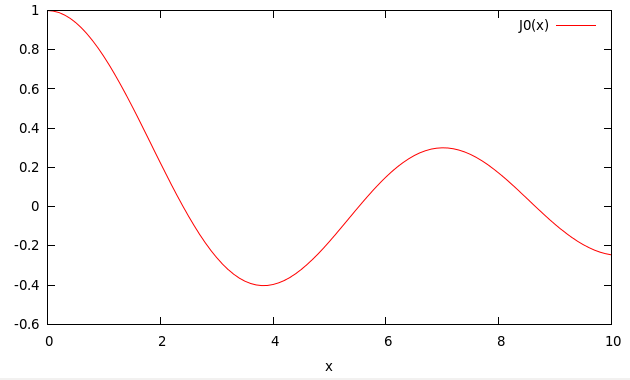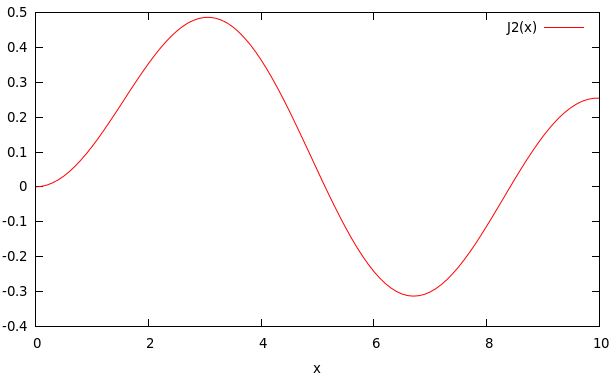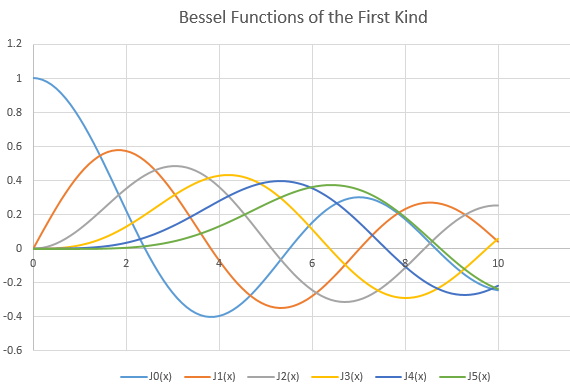# Bessel Function Series – C PROGRAM

In this post we will write a C program to calculate the Bessel Functions of the first kind.

We will use the series representation of Bessel functions, and use the concept of previous posts to calculate the sum of infinite series, and thus find the values.

The series is given as:$J_n(x)=\left(\frac{x}{2}\right)^n\sum_{k=0}^\infty\frac{(-1)^k(\frac{x^2}{4})^k}{k!(n+k)!}$

As you can notice, we would need to calculate factorials for the series sum evaluation.
We already wrote a program for that here.
So we will just use the function to calculate and return the factorial.

If you haven’t already checked out my past posts on Series Summation then you can read the following articles, before you move on with this post.
Sine Series(Finite) – C Program
Sine Series(Infinite) – C Program
Cosine Series(Infinite) – C Program

If we remove the$\left(\frac{x}{2}\right)^n$ of the definition given above, then we get the series:$S=\sum_{k=0}^\infty\frac{(-1)^k(\frac{x^2}{4})^k}{k!(n+k)!}$

So we can simply calculate the sum of this series and then multiply the result by$\left(\frac{x}{2}\right)^n$, to get the value of the Bessel Function.

The first term of the new series is:$t_0=\frac{1}{n!}$

and the ratio of consecutive terms is:$R=\frac{t_k}{t_{k-1}}=-\frac{x^2/4}{k(n+k)}$
Note: k starts from 1 for our ratio to work.

Now, that you have the above information, the Bessel Function for a given value of$n$ and, correct upto a certain number of decimal places can be easily calculated. These values can be written down to a file and then they can be plotted using a software like Gnuplot.

### PROGRAM:

/*****************************
******BESSEL FUNCTION********
***********SERIES************
****************************/
#include<stdio.h>
#include<math.h>
double factorial(int n){
int i;
double fact=1;
for(i=n;i>=1;i--){
fact=fact*i;
}
return fact;
}
main(){
FILE *fp=NULL;
fp=fopen("besselSeriesPlotn0.txt","w");
double t0,t1,R,sum,x,eps;
int n;
printf("Enter the value of n: ");
scanf("%d",&n);
printf("Enter the desired accuracy: ");
scanf("%lf",&eps);
for(x=0;x<=10;x=x+0.1){
int k=1;
//Initialize First Term
t0=1/factorial(n);
//Make sum equal to the first term
sum=t0;
do{
//Find the ratio of the second term to the first term using already known relation
R=-(x*x/4)/(k*(n+k));
//Calculate the second term
t1=R*t0;
//find the new sum
sum=sum+t1;
t0=t1;
k++;
//keep on summing terms until the required accuracy is reached
}while(fabs(t1/sum)>eps);
sum=sum*pow(x/2,n);
fprintf(fp,"%lf\t%lf\n",x,sum);
}

}


The above program will prompt the user to enter the value of$n$ and the accuracy desired, and then it will write down the data-points to the text file 'besselSeriesPlotn0.txt'.
Then this file can be plotted using Gnuplot command:
->plot 'besselSeriesPlotn0.txt' w l
Programmer, can change the name of the file to be written for different values of$n$ and then plot and see the results.

### OUTPUT:

The following are the output results for a few sample runs of the program:

#### Gnuplot Output:#### Gnuplot Output:#### Gnuplot Output:In the following image, I have plotted many Bessel functions simultaneously, by compiling the outputs for different$n$:Hope, you found this post helpful.
If you have any questions/doubts leave a comment down below.

### Related:

Sine Series(Finite) – C Program
Sine Series(Infinite) – C Program
Cosine Series(Infinite) – C Program
Problems on Series Sum – C

### References:[wpedon id="7041" align="center"]

## 4 thoughts on “Bessel Function Series – C PROGRAM”

1. what’s the command to plot using different colors??
btw,thankyou very much.was very helpful 😀

2. sir, I want to know about Inokutti-Hirayama model (IH) fitting for non-exponential curve.

1. Unfortunately, I’m not aware of it.

3. Hii, this code does not work for higher values ok x (> 500) if we increase x then J should converge but it goes to infinite instead, can you explain why?